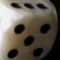# Man's physical universe296 FORMS OF ENERGY

9. What is the true nature of heat energ>?

10. List four difTerent methods for the production of heat.

11. How are the two factors of heat energy- measured?

12. State the first two laws of thermodynamics.

13. Why is a perpetual-motion machine im5X)ssible?

14. What is meant by the term "work"?

15. What is power?

16. Why is it desirable to use engines or motors which will deliver more pxjwer

than is generally required?

17. If a 10-h.p. engine and a 100-h.p. engine each had the same weight and each

one were to be placed in an identical chassis, would one engine use more

gasoline than the other engine in climbing a 10-mile grade provided only

5 h.p. were required to climb the grade? Why or why not?

18. What is a horsepower?

19. What becomes of that portion of the energy supplied to a machine that does

not do useful work?

20. How is friction eliminated in machinery?

21. Why is friction such an important problem in the construction of machinery?

22. Prepare a list of useful applications of friction.

23. Why should one use low gears rather than brakes of an automobile in descending

a long steep grade? Where is the friction produced when using low

gears? Why is the friction produced when descending in low gears less harmful

than that produced when using the brakes?

24. Classify the following examples of energy: (a) a speeding automobile, (b) a

glacier, (c) the solar s>stem, (d) hot water, (e) dynamite, (/) sugar.